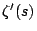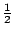# Distribution of zeros of derivatives of characteristic polynomials

The eigenvalues of a unitary matrix lie on the unit circle. Therefore the zeros of the derivative of the characteristic polynomial of the matrix lie strictly inside the unit circle (apart from those coming from multiple zeros).

How are the zeros of the derivative distributed? What is the distribution of the absolute value of those zeros?

This question is related to the question of zeros of the derivative of the zeta--function, where one would like to know how far the zeros oflie to the right of the-line.

Back to the main index for L-functions and Random Matrix Theory.